# 分类算法-NB（NaiveBeyesian Classification）分类器及AUC效果评估

1. 特征表示：X={昨日，投资，市场。。。。。。}

2. 特征选择：X={国内，投资，市场}

3. 模型选择：朴素贝叶斯分类器

4. 训练数据准备

5. 模型训练

6. 预测（分类）

7. 评估

p（yi|X）=p(X|yi)p(yi)/p(X)

P(X):待分类对象自身的概率，可忽略

P(yi):每个类别的先验概率，如P(军事)

P(X|yi):每个类别产生该对象的概率

P(xi|yi):每个类别产生该特征的概率，如P(苹果|科技)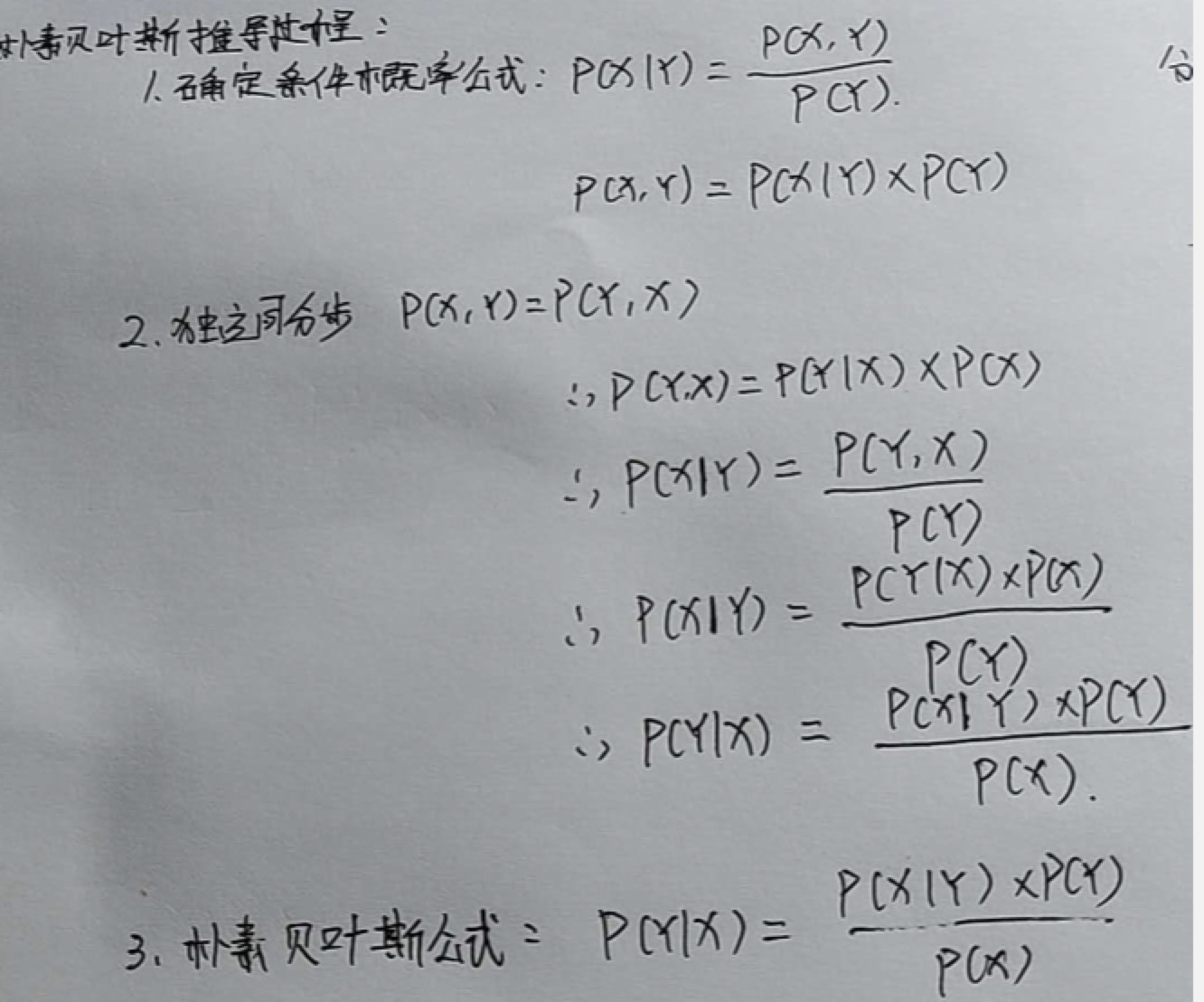P(yi)

– p(军事)=0.3， p(科技)=0.24， p(生活)=0.14，......

P(xj|yi)

– P(谷歌|军事)=0.05， P(投资|军事)=0.03， P(上涨|军事)=0.12，......

– P(谷歌|科技)=0.15， P(投资|科技)=0.10， P(上涨|科技)=0.04，......

– P(谷歌|生活)=0.08， P(投资|生活)=0.13， P(上涨|生活)=0.18，......

P(y=军事) = 50/100

P(y=财经) = 30/100

P(y=体育) = 20/100

P(X):这篇文章的概率=是一个固定值，可以忽略掉

P(yi|X)≈ P(yi)P(X|yi)

P(X|yi)：对于y指定的类别中，出现X的概率

P(xi|yi):对于y指定的类别中，出现x这个词的概率

y=军事，x=军舰

X={军舰、大炮、航母}

P(X|y=军事) = P(x=军舰|y=军事)*P(x=大炮|y=军事)*P(x=航母|y=军事)

P(yi|X)≈ P(yi)P(X|yi)

1、先验概率P(yi)

2、条件概率P(X|yi)

分子：军事类文章中包含“谷歌”这个词的文章个数

分母：军事类文章个数

p(x="谷歌"|y="军事")：分子 / 分母

分子：军事类文章中包含“谷歌”这个词的个数

分母：军事类文章中所有词的个数

p(x="谷歌"|y="军事")：分子 / 分母

import sys
import os
import random

WordList = []
WordIDDic = {}
#训练阈值
TrainingPercent = 0.8

#输入文件夹
inpath = sys.argv
#输出的文件
OutFileName = sys.argv
#输出的文件训练集是OutFileName.train,测试集是：OutFileName.test
trainOutFile = file(OutFileName+".train", "w")
testOutFile = file(OutFileName+".test", "w")

def ConvertData():
i = 0
tag = 0
for filename in os.listdir(inpath):
#只分析三大类的数据，财经，汽车，体育，并且给每个类别打个标示，财经是1，汽车2，体育3
tag = 1
elif filename.find("auto") != -1:
tag = 2
elif filename.find("sport") != -1:
tag = 3
#统计一共读了多少篇文章
i += 1
#设置一个随机数，为了下面能够把数据按照二八原则分为训练集和测试集
rd = random.random()
#把测试集的文件名赋给outfile变量
outfile = testOutFile
#若随机数小于0.8则把训练集文件名副歌outfile变量，这种操作是为了下面写入数据做准备
if rd < TrainingPercent:
outfile = trainOutFile

if i % 100 == 0:
print i,"files processed!\r",
#读入目录下的文章内容，inpath是目录地址，filename是目录下的文件名称
infile = file(inpath+'/'+filename, 'r')
#首先把三类文章的标签写入输出文件开头，后面加空格
outfile.write(str(tag)+" ")
#进行编码转义
content = content.decode("utf-8", 'ignore')
#因为一次性读入，所以会有换行的问题，这里直接把换行替换成空格，并且以空格切分开，words是个列表
words = content.replace('\n', ' ').split(' ')
#循环列表
for word in words:
if len(word.strip()) < 1:
continue
'''这里的代码是我觉得写的最好的一段代码，简单又复杂。
首先判断这个单词在不在token2Id的字典里面，若不在，将该单词添加到一个wordList列表中
然后将该单词存入token2Id的字典里面，key是word，value是wordList列表的长度
这个if判断的精华在于利用判断word是否在token2id的字典来进行去重复
'''
if word not in WordIDDic:
WordList.append(word)
WordIDDic[word] = len(WordList)
#将word对应的id写入输出文件中
outfile.write(str(WordIDDic[word])+" ")
#最后一篇文章转换完成后，用#号隔开文章名称和内容
outfile.write("#"+filename+"\n")
infile.close()

print len(WordList), "unique words found!"

#首先调用ConvertData（）函数
ConvertData()
trainOutFile.close()
testOutFile.close()


#Usage:
#Training: NB.py 1 TrainingDataFile ModelFile
#Testing: NB.py 0 TestDataFile ModelFile OutFile

import sys
import os
import math

DefaultFreq = 0.1
TrainingDataFile = "nb_data.train"
ModelFile = "nb_data.model"
TestDataFile = "nb_data.test"
TestOutFile = "nb_data.out"
ClassFreq = {}#频次 classid下token的总数
WordDic = {} #token字典
ClassFeaProb = {} #条件概率字典
ClassDefaultProb = {} #默认概率字典 默认概率分母与条件概率分母一致
ClassProb = {} #先验概率字典

def Dedup(items):
tempDic = {}
for item in items:
if item not in tempDic:
tempDic[item] = True
return tempDic.keys()

#加载数据，初始化
i =0
#读入训练集
infile = file(TrainingDataFile, 'r')
#以行的方式读入，在上次的Data处理中已经转换成每一行的内容，首先读入一行
#判断该文件是否有内容，循环所有的训练集
while len(sline) > 0:
#找到没行的#号和文章名，输出是个索引index数字
pos = sline.find("#")
if pos > 0:
#取出从该篇文章开始到#号的位置
sline = sline[:pos].strip()
#将取出的文章内容以空格分割开
words = sline.split(' ')
#判断文章有么有问题
if len(words) < 1:
print "Format error!"
break
#得到刚刚那三类文章的tag即财经是1，汽车2，体育3 赋给classid
classid = int(words)
#判断这类型文章在不在文章字典里面，不在进入if，在进行频次+1，为了求先验概率
ClassFeaProb[classid] = {}
#给当前的classid记录频次放入频次几点里面
ClassFreq[classid]  = 0
ClassFreq[classid] += 1
#获取除了文章标签的真正内容
words = words[1:]
#remove duplicate words, binary distribution
#words = Dedup(words)
#循环内容
for word in words:
if len(word) < 1:
continue
#获取每个词，这里词是我们转置的数字
wid = int(word)
#判断当前的词在不在词语字典里面，不在初始化为1
if wid not in WordDic:
WordDic[wid] = 1
#若当前词在该词的字典里面，紧接着判断当前词在不在当前classid文章中的字典里面，不在初始化为1，在的话记录频次
else:
#记录读的总行数
i += 1
#接着再读入一行知道结束
infile.close()
print len(ClassFreq), "classes!", len(WordDic), "words!"

#计算模型
def ComputeModel():
sum = 0.0
#循环遍历不同类文章记录的字典的value值，key是classid value是该类对应的频次
for freq in ClassFreq.values():
#sum将所有的value相加即得到三类文章的总的篇幅数
sum += freq
#循环遍历不同类文章记录的字典的key值，key是classid value是该类对应的频次
for classid in ClassFreq.keys():
#当前classid对应的先验概率，用体育举例子，体育类文章篇幅数除以总的篇幅数，循环计算三类文章各个先验概率
ClassProb[classid] = (float)(ClassFreq[classid])/(float)(sum)
#循环遍历每类文章中每个词频的字典中的key，key是classid，value是个字典{词：词频}
#Multinomial Distribution
sum = 0.0
#循环遍历当前classid对应的value字典{词：词频}，中的key即词
#统计当前类文章的词频次数
#newsum = (float)(sum+len(WordDic)*DefaultFreq)
#为了使程序健壮，防止向下溢出，这里可以把sum+1
newsum = (float)(sum + 1)
#Binary Distribution
#newsum = (float)(ClassFreq[classid]+2*DefaultFreq)
#循环遍历当前类文章的的key即词
#存入条件概率值，用体育举例子，体育文章中铅球的条件概率=铅球在体育文章中的总数／体育文章的总词数
#每一类文章设置一个默认的条件概率，防止在测试集时候一个词在当前类文章没有，就用该值
ClassDefaultProb[classid] = (float)(DefaultFreq) / newsum
return

'''

共四行.第一行 classid 先验概率 默认概率
第二行属于class_A tokenid 条件概率 tokenid 条件概率 tokenid 条件概率
第三行属于class_B tokenid 条件概率 tokenid 条件概率 tokenid 条件概率
第四行属于class_C tokenid 条件概率 tokenid 条件概率 tokenid 条件概率
'''
def SaveModel():
#以写的方式打开该类文件
outfile = file(ModelFile, 'w')
#循环遍历类别频次字典，获取三大类别的classid
for classid in ClassFreq.keys():
#将classid写入文件
outfile.write(str(classid))
outfile.write(' ')
#将获取的先验概率写入文件
outfile.write(str(ClassProb[classid]))
outfile.write(' ')
#将默认的每类别条件概率写入文件
outfile.write(str(ClassDefaultProb[classid]))
outfile.write(' ' )
#第一行遍历3类文章对应的不同概率，然后换行
outfile.write('\n')
#循环遍历每个类别对应的词和词频的字典，key是classid，value是{词：词频}
#循环value的key即词
#将获得的词用来获取该词的条件概率
outfile.write(str(wid)+' '+str(ClassFeaProb[classid][wid]))
outfile.write(' ')
#每一类的文章模型为一行
outfile.write('\n')
outfile.close()

#加载模型
global WordDic
WordDic = {}
global ClassFeaProb
ClassFeaProb = {}
global ClassDefaultProb
ClassDefaultProb = {}
global ClassProb
ClassProb = {}
#读入模型
infile = file(ModelFile, 'r')
#每篇文章以空格分割开
items = sline.split(' ')
if len(items) < 6:
print "Model format error!"
return
i = 0
while i < len(items):
#获取每类文章的tag
classid = int(items[i])
#定义一个字典
ClassFeaProb[classid] = {}
#将i+1
i += 1
if i >= len(items):
print "Model format error!"
return
#存放先验概率
ClassProb[classid] = float(items[i])
#i+1接下来是默认的条件概率
i += 1
if i >= len(items):
print "Model format error!"
return
#存放默认的条件概率
ClassDefaultProb[classid] = float(items[i])
#i+1 接下来就是另外一个类别的文章
i += 1
#循环遍历条件概率的key，key是不同类别的文章classid
for classid in ClassProb.keys():
#接下来读入第二行数据，就是某一类文章的某个词的条件概率
#以空格分割开
items = sline.split(' ')
i = 0
#循环遍历
while i < len(items):
#获取该词的条件概率
wid  = int(items[i])
#判断在不在词字典里，不再初始化
if wid not in WordDic:
WordDic[wid] = 1
#若在将i+1
i += 1
if i >= len(items):
print "Model format error!"
return
#并且给当前文章类的该词字典中赋上条件概率
ClassFeaProb[classid][wid] = float(items[i])
#接下来循环第二个词，以此类推
i += 1
infile.close()
print len(ClassProb), "classes!", len(WordDic), "words!"

def Predict():
global WordDic
global ClassFeaProb
global ClassDefaultProb
global ClassProb

TrueLabelList = []
PredLabelList = []
i =0
#读入测试集
infile = file(TestDataFile, 'r')
outfile = file(TestOutFile, 'w')
#以每行读入，这里是每篇文章以及文章中的词
scoreDic = {}
iline = 0
while len(sline) > 0:
#上来将iline+1，是为了从第二个开始，第一是文章标示的tag
iline += 1
if iline % 10 == 0:
print iline," lines finished!\r",
#这块和前面的一样，掠过#后面的文件名称
pos = sline.find("#")
if pos > 0:
sline = sline[:pos].strip()
#将文章的内容进行以空格切分开
words = sline.split(' ')
if len(words) < 1:
print "Format error!"
break
#获取当前文章类别
classid = int(words)
#将类别添加到标签list中
TrueLabelList.append(classid)
#内容从1开始知道最后
words = words[1:]
#remove duplicate words, binary distribution
#words = Dedup(words)
#循环遍历每个类别的先验概率，放入scoreDic字典中
for classid in ClassProb.keys():
scoreDic[classid] = math.log(ClassProb[classid])
#循环内容
for word in words:
if len(word) < 1:
continue
wid = int(word)
#过滤掉一些没有的词
if wid not in WordDic:
#print "OOV word:",wid
continue
#循环遍历当前类别的先验概率
for classid in ClassProb.keys():
#判断当前词存不存在条件概率的字典中，若不存在，直接取默认的条件概率，否则取出条件概率
if wid not in ClassFeaProb[classid]:
# 如果当前分类中不包含这个分词,就算出一个默认概率 p(x1|y) +p(x2|y) +p(x3|y) == p(军舰|军事) +p(大炮|军事)
scoreDic[classid] += math.log(ClassDefaultProb[classid])
else:
scoreDic[classid] += math.log(ClassFeaProb[classid][wid])
#binary distribution
#wid = 1
#while wid < len(WordDic)+1:
#   if str(wid) in words:
#       wid += 1
#       continue
#   for classid in ClassProb.keys():
#       if wid not in ClassFeaProb[classid]:
#           scoreDic[classid] += math.log(1-ClassDefaultProb[classid])
#       else:
#           scoreDic[classid] += math.log(1-ClassFeaProb[classid][wid])
#   wid += 1
i += 1
#取出最大的概率
maxProb = max(scoreDic.values())
#并且循环分数字典
for classid in scoreDic.keys():
if scoreDic[classid] == maxProb:
PredLabelList.append(classid)
infile.close()
outfile.close()
print len(PredLabelList),len(TrueLabelList)
return TrueLabelList,PredLabelList
#计算准确率
def Evaluate(TrueList, PredList):
accuracy = 0
i = 0
while i < len(TrueList):
if TrueList[i] == PredList[i]:
accuracy += 1
i += 1
accuracy = (float)(accuracy)/(float)(len(TrueList))
print "Accuracy:",accuracy
'''
计算精确率和召回率:
精确率:针对军事分类,  预测军事成功的个数/预测为军事的总数
召回率:针对军事分类,  预测军事成功的个数/实际军事类文章的个数
'''
def CalPreRec(TrueList,PredList,classid):
correctNum = 0
allNum = 0
predNum = 0
i = 0
while i < len(TrueList):
if TrueList[i] == classid:
allNum += 1
if PredList[i] == TrueList[i]:
correctNum += 1
if PredList[i] == classid:
predNum += 1
i += 1
return (float)(correctNum)/(float)(predNum),(float)(correctNum)/(float)(allNum)

#main framework
'''代码执行的主要部分
判断输入参数，是训练集还是测试集，当然，我们拿到数据首先进行训练，然后进行测试，最后的出结果
那么我们一个一个来看下
'''
if len(sys.argv) < 4:
print "Usage incorrect!"
#当参数是1的时候进入训练集
elif sys.argv == '1':
print "start training:"
TrainingDataFile = sys.argv  # 训练数据 tag 自增长的id 自增长的id
ModelFile = sys.argv  # model模型数据输出文件
ComputeModel()  # 计算先验概率ClassProb 和条件概率 ClassFeaProb
SaveModel()
#模型训练完成，开始进行测试
elif sys.argv == '0':
print "start testing:"
TestDataFile = sys.argv
ModelFile = sys.argv
TestOutFile = sys.argv

#通过测试集与模型计算，获得classid和每篇文章的分类结果
TList,PList = Predict()
i = 0
outfile = file(TestOutFile, 'w')
#循环取出每篇文章以及对应的分类
while i < len(TList):
outfile.write(str(TList[i]))
outfile.write(' ')
outfile.write(str(PList[i]))
outfile.write('\n')
i += 1
outfile.close()
Evaluate(TList,PList)
for classid in ClassProb.keys():
pre,rec = CalPreRec(TList, PList,classid)
print "Precision and recall for Class",classid,":",pre,rec
else:
print "Usage incorrect!"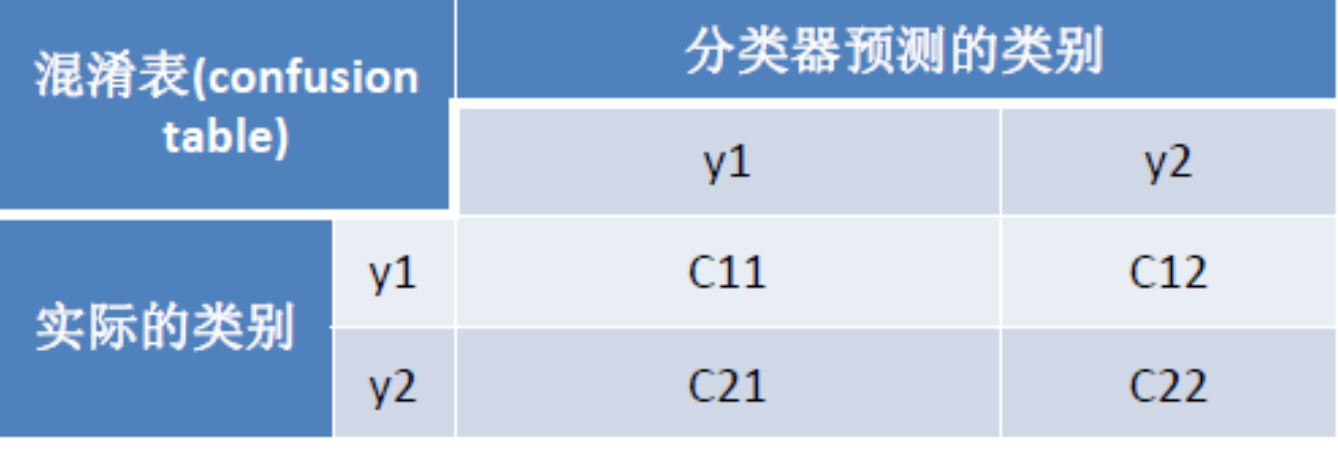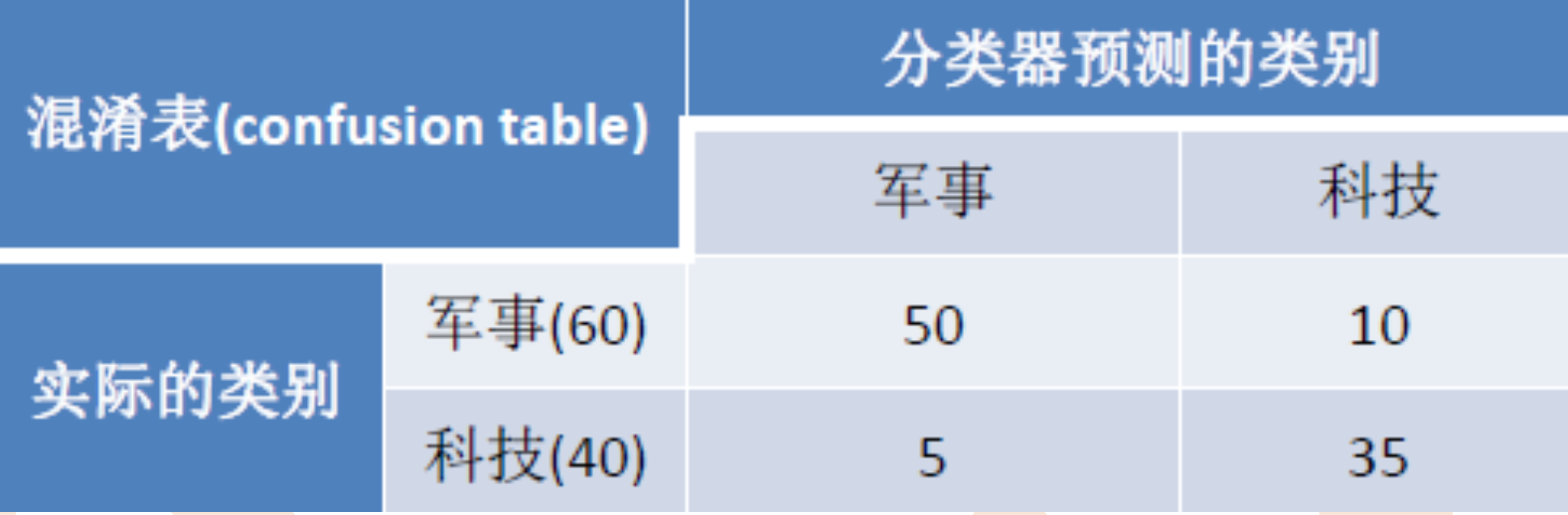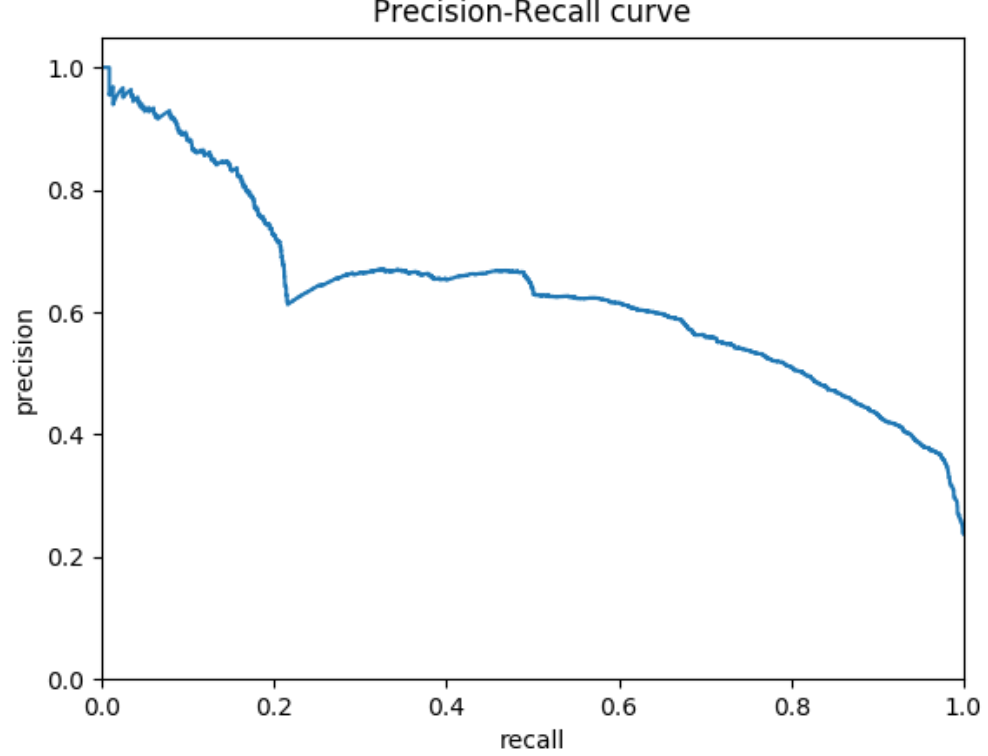1. 搜索场景，保证召回的前提下，再提高准确率

2. 疾病检测，保证准确性前提，提升召回

PR曲线是个评测指标，用来协助你去选择阀值的，那么我们看下ROC曲线的评测指标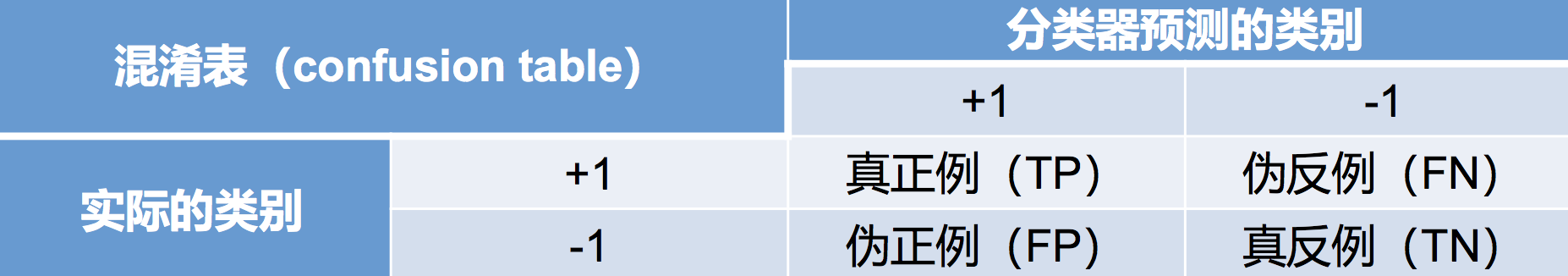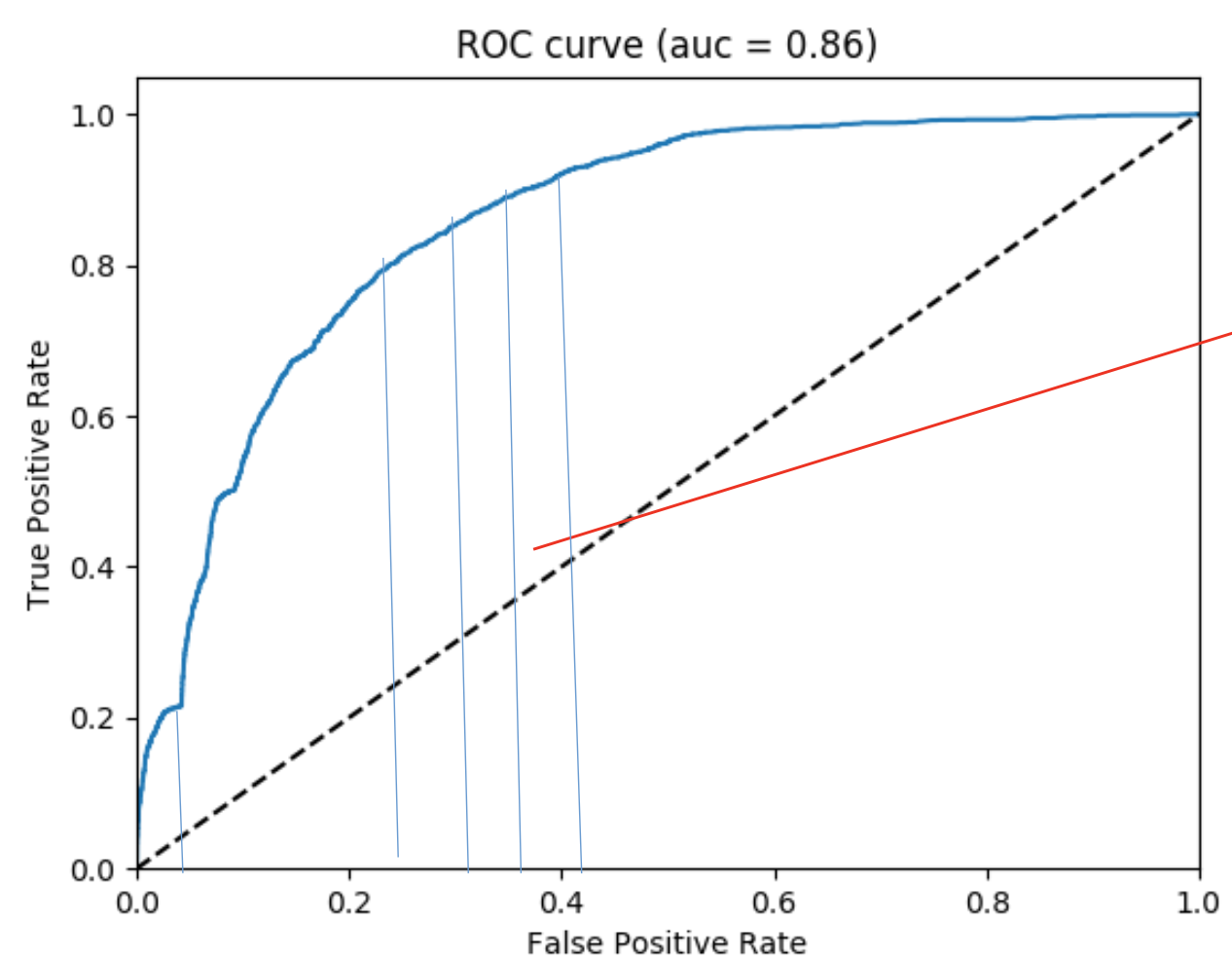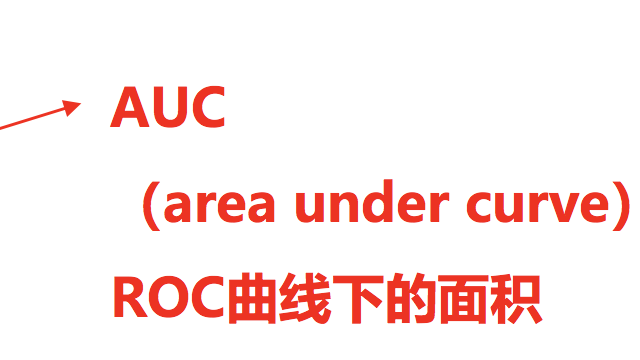cat auc.raw | sort -t$'\t' -k2g |awk -F'\t' '($1==-1){++x;a+=y;}(\$1==1){++y;}END{print 1.0-a/(x*y);}'

x*y是正负样本pair对，a代表错误的个数，a/x*y 错误的概率，1-a/x*y 正确概率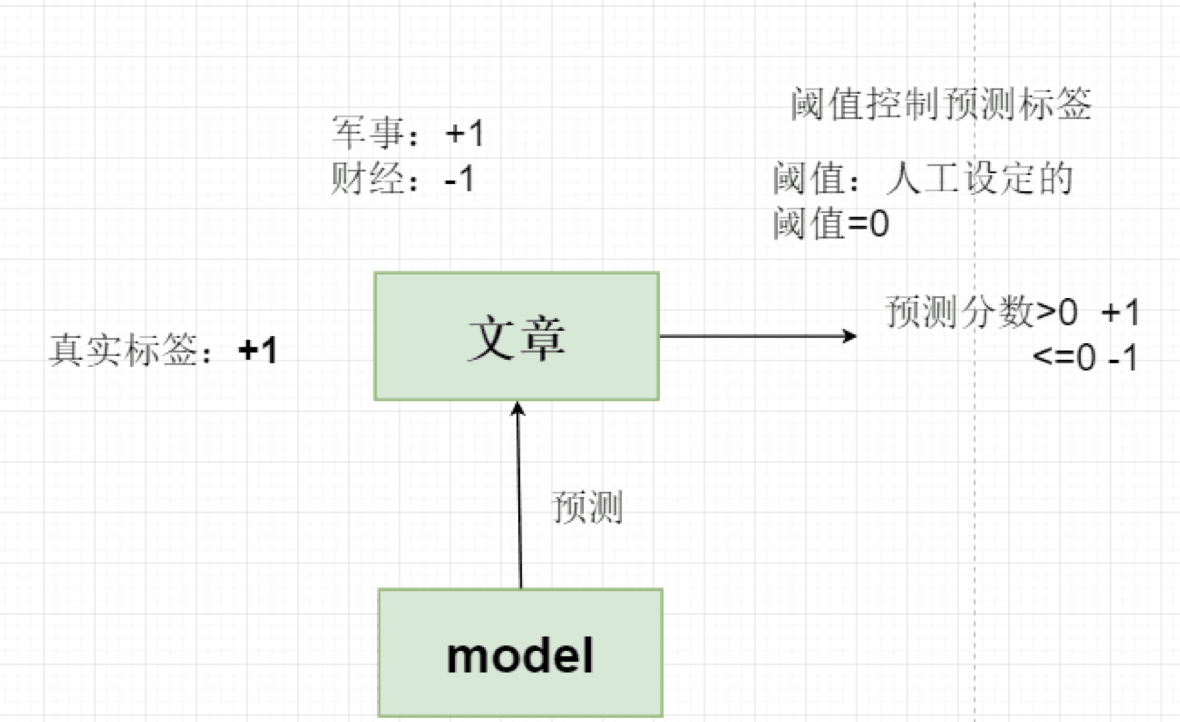04-191029

#### Naive Bayes算法（朴素贝叶斯算法）03-01615

#### 朴素贝叶斯（NaiveBeyesian Classification，NB）

04-2513万+

#### 总结了 150 余个神奇网站，你不来瞅瞅吗？©️2020 CSDN 皮肤主题: 技术黑板 设计师: CSDN官方博客点击重新获取扫码支付1.余额是钱包充值的虚拟货币，按照1:1的比例进行支付金额的抵扣。
2.余额无法直接购买下载，可以购买VIP、C币套餐、付费专栏及课程。余额充值